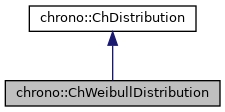chrono::ChWeibullDistribution Class Reference

## Description

Class that generates the Weibull distribution.

It can be used for example to describe particle size distribution, as in the subcase of Rosin & Rammler distribution.

#include <ChDistribution.h>

Inheritance diagram for chrono::ChWeibullDistribution:[legend]
Collaboration diagram for chrono::ChWeibullDistribution:[legend]

## Public Member Functions

ChWeibullDistribution (double mlambda, double mk)
Create the Weibull distribution with assigned scale factor 'lambda' and with shape 'k'. More...

virtual double GetRandom () override
Compute a random value whose probability density is the Weibull distribution. More...

double GetK () const

double GetLambda () constPublic Member Functions inherited from chrono::ChDistribution
virtual ~ChDistribution ()
Default destructor for distribution object.

## Constructor & Destructor Documentation

 chrono::ChWeibullDistribution::ChWeibullDistribution ( double mlambda, double mk )

Create the Weibull distribution with assigned scale factor 'lambda' and with shape 'k'.

The larger 'lambda' is, the more horizontally stretched is the distribution. For k<1, there is a vertical peak at 0. For k=1, you get the exponential distribution. For k>1, you get an asymmetric bell shape.

## Member Function Documentation

 double chrono::ChWeibullDistribution::GetRandom ( )
overridevirtual

Compute a random value whose probability density is the Weibull distribution.

It uses the "Smirnov transform" (inverse probability integral transform)

Implements chrono::ChDistribution.